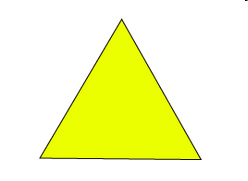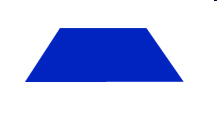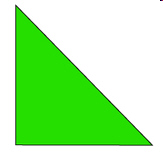6.G.1. Test 1 B
 Name:    6.G.1. Test 1 B

Multiple Choice
Identify the choice that best completes the statement or answers the question.

1.

height = 8ft   base = 8ft

What is the area of this triangle?a. 16 sq ft c. 8 sq ft b. 32 sq ft d. 64 sq ft

2.b1= 10 in.   b2 = 6 in  h = 5

Find the area of the trapezoid.
 a. A = 150 sq in c. A = 300 sq in b. A = 40 sq in d. A = 80 sq in

3.S = 8 cm

What is the area of this square?
 a. 16 sq cm c. 32 sq cm b. 64 sq cm d. 8 sq cm

4.w = 4 mm         l = 6 mm

What is the area of the rectangle?
 a. 6 sq mm c. 12 sq mm b. 20 sq mm d. 24 sq mm

5.b = 8mm    A = 40 mm2

What is the measurement of the height?
 a. 10 mm c. 16 mm b. 5 mm d. 4 mm